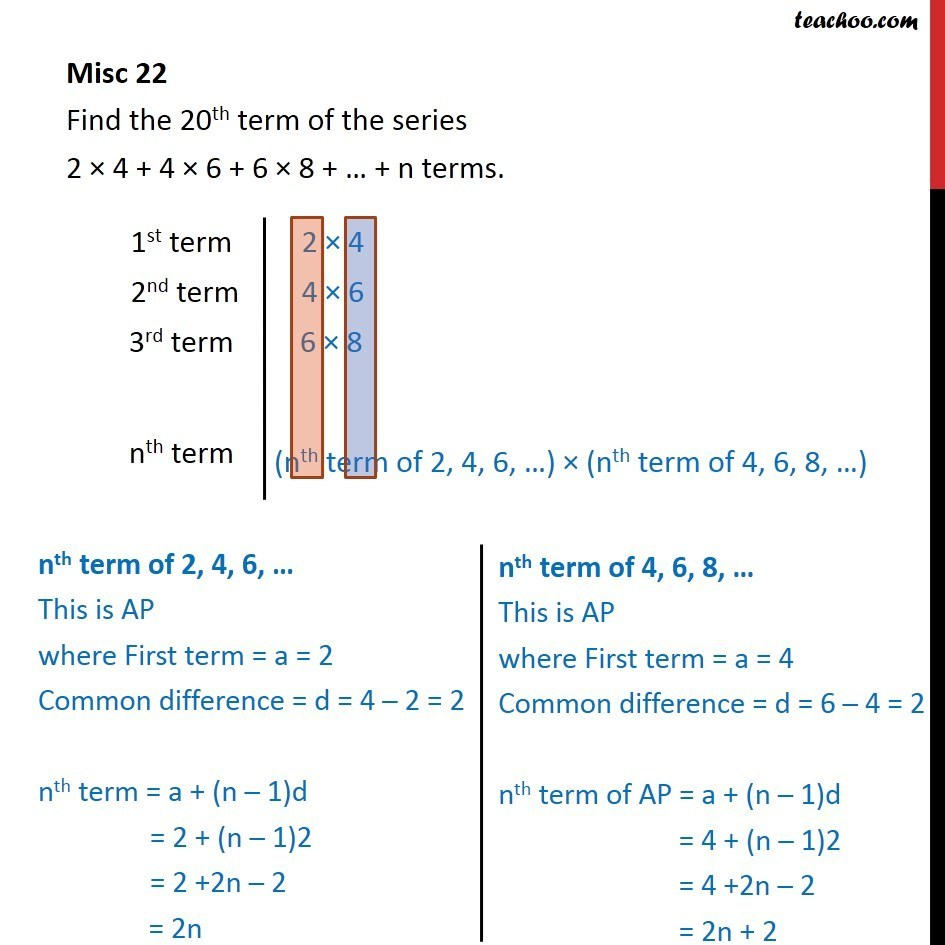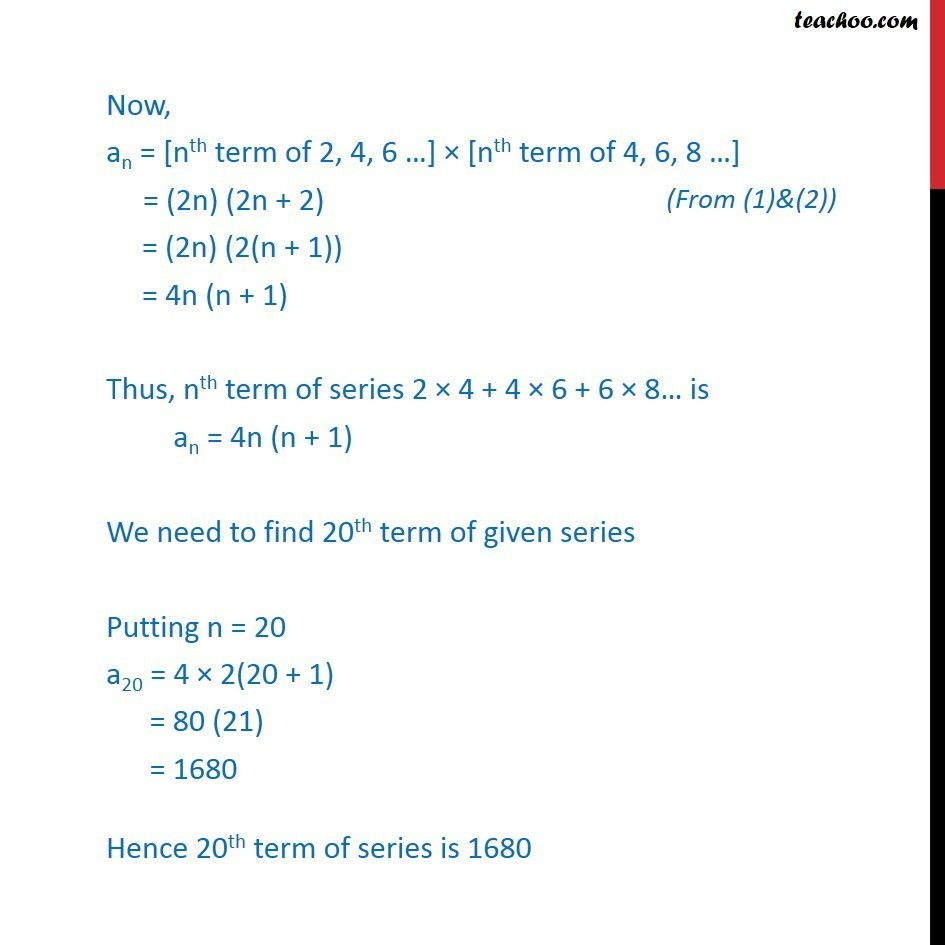Finding sum from nth number

Chapter 8 Class 11 Sequences and Series
Concept wiseLearn in your speed, with individual attention - Teachoo Maths 1-on-1 Class

### Transcript

Misc 12 Find the 20th term of the series 2 × 4 + 4 × 6 + 6 × 8 + … + n terms. Now, an = [nth term of 2, 4, 6 …] × [nth term of 4, 6, 8 …] = (2n) (2n + 2) = (2n) (2(n + 1)) = 4n (n + 1) Thus, nth term of series 2 × 4 + 4 × 6 + 6 × 8… is an = 4n (n + 1) We need to find 20th term of given series Putting n = 20 a20 = 4 × 2(20 + 1) = 80 (21) = 1680 Hence 20th term of series is 1680